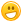# FOR ALL DEM MATH WHIZZES OUT DER...

816 views
asked Aug 25, 2015 in Math
reshown Aug 30, 2015

WHAT'S THE ANSWER TO THESE PROBLEMS?

1. 3X_=1+2=_?

2.4+1=_X6=_?

3.22-__=20+1x1=_?

WHOEVER GETS THEM DONE FIRST WHENS MY HONORARY KIDZSEARCH MATH-WHIZ AWARD.

+1 vote
answered Aug 17, 2016 by Sparkle101
selected Aug 18, 2016 by rae

I dont know them
commented Aug 18, 2016 by (87,410 points)

It's OK I wrote order of operations wrong anyway!answered Aug 30, 2015 by (87,410 points)

Whizkid, The answers are (in order)

3

30

21

Actually SIMPLIFY instead of making nothing out of it. I don't want a lecture, if I did, i'd disobey someone. so get it together and READ IT. that's all u had to do.

commented Aug 30, 2015 by (65,700 points)
No clue how those answers are right, but will take your word on it...
commented Nov 12, 2016 by (38,060 points)
Im not tryin to be mean or anything,

whizkid, maybe you could try to

OPEN UR MIND A BIT
commented Nov 12, 2016 by (65,700 points)

I just don't understand the question, sorry ...

22-__=20+1x1=_?

I see how 20 + 1x1 = 21

But the first part, 22 - ___ would not be right with 21 as the __ answer, since 22 - 21 = 1

commented Nov 14, 2016 by (38,060 points)
My math teacher always says that to do math efficiently:

You don't always have to try to make a big deal and try to calculate the answer.

She says:

"Use what operations u already know. (Like x, +, - like those.)"

See what I mean?
answered Aug 26, 2015 by (65,700 points)
1.  3X_=1+2=_?
The initial question does not really make total sense, since it is say 3 times some number equals 3, which also equals that same number. If I let y = "some number"

3Xy=1+2= y
3Xy=3=y
dividing by y
3 = 3/y = 1
There is no answer, since y=3 will make the right part correct, but not the left side.

I *think* the question is asking  3X_=1+2, solve for _
Letting y=_
3Xy=1+2
3Xy=3
Divide each side by 3
1Xy=1
y=1
_=1

2.  4+1=_X6=_?

4 + 1 = yX6
5=yX6
divide each side by 6
5/6 = yX1
y = 5/6

3.  22-__=20+1X1=_?

22 - y = 20 + 1X1
simplify right side
22 - y = 20 + 1
22 - y = 21
subtract 22 from both sides
(22 - 22) - y = 21 - 22
0 - y  = -1
-y = -1
divide both sides by -1 to remove the -1 sign
-y/-1 = -1/-1
y = 1